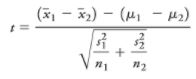# Hypothesis Testing

We use statistics to answer difficult questions, often about the future. For example, If I do this, then that will happen. We use educated guesses like this, or hypotheses, to make better decisions. Hypothesis testing is the statistical process we use to test a hypothesis to see if the answer or results we get are significant or simply due to random chance. If the answer to a question or hypothesis we test is significant, then we can use that answer with confidence to help us make decisions about what to do and how it will affect the future.

## Here are the basic steps to a hypothesis test:

1. Set up the null & alternative hypothesis
2. Decide the significance level you will use to reject or fail to reject the null hypothesis (i.e. 10%, 5%, 1%)
3. Calculate your test statistic (z-statistic, t-statistic, etc.)
4. Find the critical value or P-value
5. Reject or fail to reject the null hypothesis and interpret the results

Let’s walk through the steps of hypothesis testing with an example.

Have you ever seen a bullfrog? The North American bullfrog is one of the most invasive amphibians in the world. Bullfrogs are not native to the western US, but the bullfrog population in the western US is booming. Why? Because a single adult female bullfrog can lay about 20,000 eggs and bullfrogs can eat just about anything including their own kind. Yes, they’re cannibals. Alligators, large water snakes, and snapping turtles prey on bullfrogs in their native habitat in the eastern US and help to regulate the bullfrog population, but bullfrogs have no real predators in the western US.

Let’s pretend we are scientists in Northern California studying ways we can control the bullfrog population. We’ve heard about other scientists who have had success controlling bullfrog populations by introducing Dragonfly nymphs that like to eat bullfrog tadpoles. We want to run an experiment to learn if Dragonfly nymphs could help us control the bullfrog population in Northern California.

We identify 30 similar ponds with bullfrog populations in Northern California. We know it takes 2-3 years before a tadpole transforms into a newly mature bullfrog of about 2.5 inches long that lives on the land rather than in the water. We randomly choose fifteen ponds and introduce Dragonfly nymphs in them. The remaining ponds will not have Dragonfly nymphs introduced in them and we will refer to them as the control group.

## 3. Three years later, we count the number of newly mature bullfrogs in each of the 30 ponds.

The mean number of newly mature bullfrogs from the 15 ponds without the introduction of Dragonfly nymphs was 64 with a standard deviation of 8. In the pond where we introduced Dragonfly nymphs, there were 40 newly mature bullfrogs with a standard deviation of 5. Is this lower number significant? That is, do we believe this lower number is the result of introducing Dragonfly nymphs? Or could it just be chance that the number was lower in this pond than the average number in the other ponds? To understand this, we first have to calculate a test statistic for a one-sided two sample t-test for means.

The first thing we do is calculate the t-test statistic with this formula:Since we don’t know the population standard deviation for each group, we’ll use a t-test. And since we don’t know the true population mean of the number of newly mature bullfrogs in a pond, we’ll use the sampling distribution of means and the standard error to calculate our test statistic.

***calculate t-statistic = -9.85

## 4. We use the t-statistic to find our P-value.

The P-value helps us understand how significant the results of our test pond are. That is, if we conclude the Dragonfly nymphs significantly decrease the bullfrog population and then repeat this experiment, how likely it would be that we get results that lead us to the opposite conclusion? The p-value for the test statistic -9.85 (P(t) < -9.85) is less than 0.0001. You can get an estimate for this number using a t-table, but feel free to use a graphing calculator (or whatever technology your instructor allows) for an exact answer.

Since our p-value is less than 0.05, this tells us that the result we found in the pond we tested with Dragonfly nymphs was significant at the 0.05 level we determined earlier. Or another way to think about it is: If we counted frogs in 100 more ponds without adding Dragonfly nymphs we would find 0.0001 or less (not that you can have 0.0001 of a pond!) with a number of frogs as low or lower than the pond we tested. As a result, the lower number of frogs in the pond we tested is significant and likely the effect of the Dragonfly nymphs we added rather than just due to random chance.

## 5. We reject the null hypothesis.

Since our test ponds had a p-value of less than 0.05 and we needed a significance level of 5% or lower, we can reject the null hypothesis that says Dragonfly nymphs do not have an effect on the number of newly mature bullfrogs.

We can conclude that our test provides sufficient evidence that introducing Dragonfly nymphs can help lower the number of bullfrog eggs that survive and become newly mature bullfrogs.

if (isMyPost) { }